# Gradient Boosted Tree Regression — Spark & Python

## An overview of GBTR using pyspark & databricks — Machine Learning

This story demonstrates the implementation of a “gradient boosted tree regression” model using python & spark machine learning. The dataset used is “bike rental info” from 2011–2012 in the capital bike share system. Our goal is to predict the count of bike rentals.

# 1. Load the data

The data in store is a CSV file. We are to create a spark data frame containing the bike data set. We cache this data so that we read it only once from the disk.

`#load the dataset & cachedf = spark.read.csv("/databricks-datasets/bikeSharing/data-001/hour.csv", header="true", inferSchema="true")df.cache()df.cache()#view the imported datasetdisplay(df)`

## Output: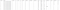Image by Author

# 2. Pre-Process the data

Fields such as “weekday” are indexed, and all the other fields except date “dteday” are numerical. The count is our target "label". The “cnt” column we aim to predict equals the sum of the “casual” & “registered” columns.

The next steps involve removing the “casual” and “registered” columns from the dataset to make sure we do not use them in predicting “cnt”. So, we discard the “dteday” and use the columns “season”, “yr”, “mnth” and “weekday”.

`#drop the features mentioneddf = df.drop("instant").drop("dteday").drop("casual").drop("registered")#print the schema of our dataset to see the type of each columndf.printSchema()`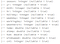Image by Author

# 3. Cast Data types

The DataFrame uses string categories, and we know that the columns are numerical in nature. So we cast them in order to proceed.

`# casts all columns to a numeric typefrom pyspark.sql.functions import col  # for indicating a column using a string in the line belowdf = df.select([col(c).cast("double").alias(c) for c in df.columns])df.printSchema()`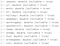Image by Author

# 4. Train & Test Sets

The data prep step splits the dataset into train and test sets. We train/tune the model on the training set.

`# Split 70% for training and 30% for testingtrain, test = df.randomSplit([0.7, 0.3])print("We have %d training examples and %d test examples." % (train.count(), test.count())`

There are 12160 training samples & 5219 test samples.

# 5. Machine Learning Pipeline

Since the data is prepared, let’s learn the ML model to predict rentals for the future.

For every row in the data, feature vectors should describe what we know: such as the weather, week(day), etc., & the label is generally what we aim to predict, in this case — (“cnt”).

We then put a Pipeline with the stages mentioned:

• VectorAssembler: This assembles feature columns into a feature vector.
• VectorIndexer: This identifies columns that are meant to be categorical heuristically, and identifies any column with a small number of distinct values as being categorical.
• GBTRegressor: This uses the (GBT) algorithm to learn & predict rental aggregates from feature vectors.
• CrossValidator: The GBT algorithm & it’s parameters, are tuned to improve accuracy of our models.
`from pyspark.ml.feature import VectorAssembler, VectorIndexerfeaturesCols = df.columnsfeaturesCols.remove('cnt')# Concatenates all feature columns into a single feature vector in a new column "rawFeatures"vectorAssembler = VectorAssembler(inputCols=featuresCols, outputCol="rawFeatures")# Identifies categorical features and indexes themvectorIndexer = VectorIndexer(inputCol="rawFeatures", outputCol="features", maxCategories=4)`

Next, we define training stage of the Pipeline. GBTRegressor takes in vectors of the features and the labels as input in order to learn to predict the target labels of newer samples.

`from pyspark.ml.regression import GBTRegressor# Takes the "features" column and learns to predict "cnt"gbt = GBTRegressor(labelCol="cnt")`

We then use cross validation to tune the parameters & achieve the best results. It trains multiple models, chooses the best, minimizing a metric. Our metric is Root Mean Squared Error (RMSE).

`from pyspark.ml.tuning import CrossValidator, ParamGridBuilderfrom pyspark.ml.evaluation import RegressionEvaluator# Define a grid of hyperparameters to test:#  - maxDepth: max depth of each decision tree in the GBT ensemble#  - maxIter: iterations, i.e., number of trees in each GBT ensemble# In this example notebook, we keep these values small.  In practice, to get the highest accuracy, you would likely want to try deeper trees (10 or higher) and more trees in the ensemble (>100)paramGrid = ParamGridBuilder()\  .addGrid(gbt.maxDepth, [2, 5])\  .addGrid(gbt.maxIter, [10, 100])\  .build()# We define an evaluation metric.  This tells CrossValidator how well we are doing by comparing the true labels with predictions.evaluator = RegressionEvaluator(metricName="rmse", labelCol=gbt.getLabelCol(), predictionCol=gbt.getPredictionCol())# Declare the CrossValidator, which runs model tuning for us.cv = CrossValidator(estimator=gbt, evaluator=evaluator, estimatorParamMaps=paramGrid)`

Lastly, we tie our features & model training together into one Pipeline.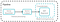Image by Author
`from pyspark.ml import Pipelinepipeline = Pipeline(stages=[vectorAssembler, vectorIndexer, cv])`

# 6. Train & Test the Pipeline

`pipelineModel = pipeline.fit(train)`

MLlib will allow trials in MLflow. After tuning fit() call is done, the MLflow UI can be accessed to view the logged runs.

`predictions = pipelineModel.transform(test)display(predictions.select("cnt", "prediction", *featuresCols))`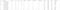Image by Author

The result may not be the best, but that’s where model tuning kicks in.

The (RMSE) mentioned above, tells us how well our model predicts on new samples.

Lower the RMSE, the better.

`rmse = evaluator.evaluate(predictions)print("RMSE on our test set: %g" % rmse)`

RMSE of the test set: 44.6918

# 7. Tips on improving the model

There are several ways we could further improve our model:

• Expert knowledge
• Better Tuning
• Feature Engineering

Different combinations of the hyperparameters are used to find the best solution.

Connect on LinkedIn and check out my Github for the complete notebook.

Data Scientist @ Mindcurv | Machine Learning Graduate | Masters in Information Systems @ Monash University | https://github.com/ranjithhmenon

## More from Ranjith Menon

Data Scientist @ Mindcurv | Machine Learning Graduate | Masters in Information Systems @ Monash University | https://github.com/ranjithhmenon